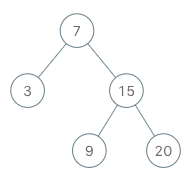# 173. Binary Search Tree Iterator

Medium
Implement the `BSTIterator` class that represents an iterator over the in-order traversal of a binary search tree (BST):
• `BSTIterator(TreeNode root)` Initializes an object of the `BSTIterator` class. The `root` of the BST is given as part of the constructor. The pointer should be initialized to a non-existent number smaller than any element in the BST.
• `boolean hasNext()` Returns `true` if there exists a number in the traversal to the right of the pointer, otherwise returns `false`.
• `int next()` Moves the pointer to the right, then returns the number at the pointer.
Notice that by initializing the pointer to a non-existent smallest number, the first call to `next()` will return the smallest element in the BST.
You may assume that `next()` calls will always be valid. That is, there will be at least a next number in the in-order traversal when `next()` is called.
Example 1:Input
["BSTIterator", "next", "next", "hasNext", "next", "hasNext", "next", "hasNext", "next", "hasNext"]
[[[7, 3, 15, null, null, 9, 20]], [], [], [], [], [], [], [], [], []]
Output
[null, 3, 7, true, 9, true, 15, true, 20, false]
Explanation
BSTIterator bSTIterator = new BSTIterator([7, 3, 15, null, null, 9, 20]);
bSTIterator.next(); // return 3
bSTIterator.next(); // return 7
bSTIterator.hasNext(); // return True
bSTIterator.next(); // return 9
bSTIterator.hasNext(); // return True
bSTIterator.next(); // return 15
bSTIterator.hasNext(); // return True
bSTIterator.next(); // return 20
bSTIterator.hasNext(); // return False
Constraints:
• The number of nodes in the tree is in the range `[1, 10^5]`.
• `0 <= Node.val <= 10^6`
• At most `105` calls will be made to `hasNext`, and `next`.
• Could you implement `next()` and `hasNext()` to run in average `O(1)` time and use `O(h)` memory, where `h` is the height of the tree?

### 解題

/**
* Definition for a binary tree node.
* type TreeNode struct {
* Val int
* Left *TreeNode
* Right *TreeNode
* }
*/
type BSTIterator struct {
arr []int
}
func Constructor(root *TreeNode) BSTIterator {
arr := make([]int, 0)
if root == nil {
return
}
arr = append(arr, root.Val)
}
return BSTIterator{arr: arr}
}
func (this *BSTIterator) Next() int {
if len(this.arr) == 0 {
return -1
}
res := this.arr
this.arr = this.arr[1:]
return res
}
func (this *BSTIterator) HasNext() bool {
if len(this.arr) == 0 {
return false
}
return true
}
/**
* Your BSTIterator object will be instantiated and called as such:
* obj := Constructor(root);
* param_1 := obj.Next();
* param_2 := obj.HasNext();
*/